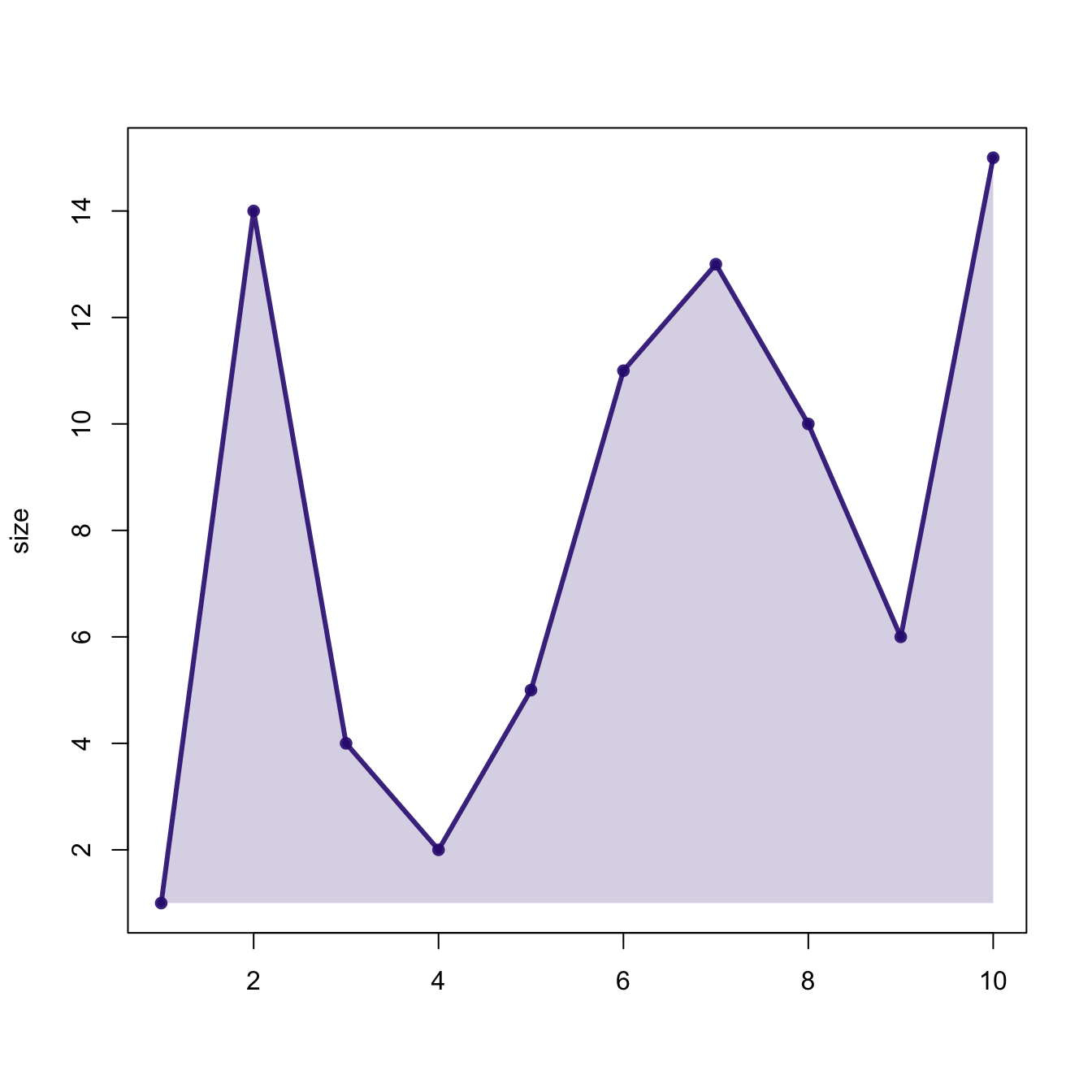# Area chart with base R

This post describes how to build an area chart using base R and the `polygon()` function. See the area chart section for a `ggplot2` implementation.

# Basic line chart with `ggplot2` and `geom_line()`

Base R also allows to build area charts thanks to the `polygon()` function. This functions requires 2 inputs: `x` and `y`.

Note that extreme values of both are added at the beginning and at the end of each vectors to make sure the polygon is closed.``````# Create data
data <- data.frame(
x=seq(1,10),
y=sample(seq(1,15),10)
)

plot( data\$x , data\$y , col=rgb(0.2,0.1,0.5,0.9) , type="o" , lwd=3 , xlab="" , ylab="size" , pch=20)

# Fill the area
polygon(
c(min(data\$x), data\$x , max(data\$x)) ,
c( min(data\$y) , data\$y , min(data\$y)) ,
col=rgb(0.2,0.1,0.5,0.2) , border=F
)``````

Related chart types

## Contact

This document is a work by Yan Holtz. Any feedback is highly encouraged. You can fill an issue on Github, drop me a message on Twitter, or send an email pasting yan.holtz.data with gmail.com.